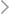International Conference on Applied Mathematics, Modelling and Intelligent Computing (CAMMIC 2021)
HomeProf. Jiansheng Geng

## Prof. Jiansheng GengProf. Jiansheng Geng

Department of Mathematics, Nanjing University, China

Speech Title：Linearly Stable KAM Tori for One Dimensional Forced Kirchhoff Equations

Abstract

We prove an abstract infinite dimensional KAM theorem, which could be applied to prove the existence and linear stability of small-amplitude  quasi-periodic solutions for one dimensional forced Kirchhoff equations with Dirichlet boundary conditions

$u_{tt}-(1+\int_{0}^{\pi} |u_x|^2 dx)u_{xx}+M_\xi u+\epsilon g(\bar{\omega}t,x) =0,\quad u(t,0)=u(t,\pi)=0,$

where $M_\xi$ is a real Fourier multiplier, $g(\bar{\omega}t,x)$ is real analytic and odd in $x$ with forced Diophantine frequencies $\bar\omega\in \R^{\nu}$, $\epsilon$ is a small parameter.

The proof is based on an improved Kuksin lemma and the off-diagonal decay property of the forcing term.

Biography

2004.6,PhD degree,Nanjing University

2006.10-2010.12 Associate Professor,Nanjing University

2010.12-present Professor, Nanjing University

The research interest: Hamiltonian Dynamical Systems